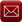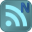You have downloaded this file 0 times in the last 24 hours, limit is 100.

## SageMath Note Book This location is for Registered Users Only.Perhaps you need to login or register.

Description:

Sign up for an account here http://www.sagenb.org/ and you can access the knowledge of http://www.sagemath.org/ to solve all your difficult maths problems.

Screencasts – videos explain how Sage works
Sage for Newbies – book by T. Kosan

When you have an account you'll be able to view lots of published calculations on http://www.sagenb.org/pub/.  If you see the error "It looks like jsMath failed to set up properly (error code -7). I will try to keep going, but it could get ugly.", you haven't installed the TeX fonts which help jsMath render beautiful mathematics. To get the nice TeX display with jsMath, please download a set of fonts from here: http://www.math.union.edu/~dpvc/jsMath/download/jsMath-fonts.html.

This free open source resource offers you the power of a full symbolic maths package like Maple, Mathematica, Matlab, and Magma (which cost \$1000 - \$2000). The browser text interface makes it easy to copy and paste into your Excel worksheets. I barely scratch the surface in terms of its power but I'll be returning to solve the following types of problem:

# Solving Equations

The `solve` function solves equations. To use it, first specify some variables; then the arguments to `solve` are an equation (or a system of equations), together with the variables for which to solve:
```sage: x = var('x')
sage: solve(x^2 + 3*x + 2, x)
[x == -2, x == -1]
```

You can solve equations for one variable in terms of others:

```sage: x, b, c = var('x b c')
sage: solve([x^2 + b*x + c == 0],x)
[x == (-sqrt(b^2 - 4*c) - b)/2, x == (sqrt(b^2 - 4*c) - b)/2]
```

You can also solve for several variables:

```sage: x, y = var('x, y')
sage: solve([x+y==6, x-y==4], x, y)
[[x == 5, y == 1]]
```

# Differentiation, Integration, etc.

Sage knows how to differentiate and integrate many functions. For example, to differentiate sin(u) with respect to u, do the following:

```sage: u = var('u')
sage: diff(sin(u), u)
cos(u)
```

To compute the fourth derivative of sin(x2):

```sage: diff(sin(x^2), x, 4)
16*x^4*sin(x^2) - 12*sin(x^2) - 48*x^2*cos(x^2)
```

To compute the partial derivatives of x2 + 17y2 with respect to x and y, respectively:

```sage: x, y = var('x,y')
sage: f = x^2 + 17*y^2
sage: f.diff(x)
2*x
sage: f.diff(y)
34*y
```

# Solving Differential Equations

You can use Sage to investigate ordinary differential equations. To solve the equation x'+x-1=0:
```sage: t = var('t')    # define a variable t
sage: x = function('x',t)   # define x to be a function of that variable
sage: DE = lambda y: diff(y,t) + y - 1
sage: desolve(DE(x(t)), [x,t])
'%e^-t*(%e^t+%c)'
```
This uses Sage's interface to Maxima [Max], and so its output may be a bit different from other Sage output. In this case, this says that the general solution to the differential equation is x(t) = et(et + c).

You can compute Laplace transforms also; the Laplace transform of t2et - sin(t) is computed as follows:

```sage: s = var("s")
sage: t = var("t")
sage: f = t^2*exp(t) - sin(t)
sage: f.laplace(t,s)
2/(s - 1)^3 - 1/(s^2 + 1)
```

# Three-Dimensional Plots

Sage produces three-dimensional plots using an open source package called [Jmol]. E.g. a twisted torus:

```sage: u, v = var('u,v')
sage: fx = (3+sin(v)+cos(u))*cos(2*v)
sage: fy = (3+sin(v)+cos(u))*sin(2*v)
sage: fz = sin(u)+2*cos(v)
sage: parametric_plot3d([fx, fy, fz], (u, 0, 2*pi), (v, 0, 2*pi),
...   frame=False, color="red")
```
Submitted By:
Submitted On:
10 Feb 2009
95
File Version:
1.0
Rating:Total Votes:1Email (preferred method of contact)US +1 617 5008224EU +44 113 8152220